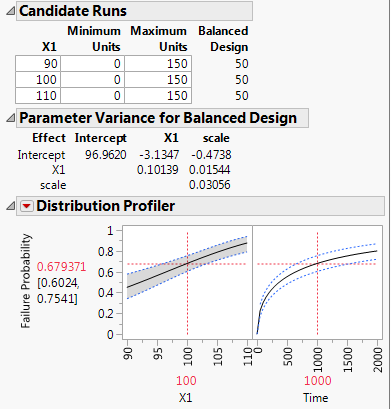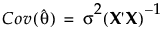Design of Experiments Guide > Accelerated Life Test Designs > Accelerated Life Test Plan Window > Review Balanced Design Diagnostics and Update Specifications
Publication date: 08/13/2020

## Review Balanced Design Diagnostics and Update Specifications

After you specify the design details and click Continue, three new outlines are added to the window.Candidate Runs

Enter the minimum and maximum number of units allowed at each level of the acceleration factors. If you are augmenting a previous experiment, for each setting, include the number of units already run at each level in the Minimum Units column.

Parameter Variance for Balanced Design

Gives a matrix proportional to the covariance matrix for the estimates of the acceleration model parameters for the balanced design.

Denote the matrix of first partial derivatives of the model with respect to the parameters, θ, by X. Denote the error variance by σ2. Under general conditions, the least squares estimator of θ is asymptotically unbiased with asymptotic covariance matrix as follows:The Parameter Variance for Balanced Design outline gives (X‘X)-1, where derivatives are calculated numerically. The calculation assumes that the values specified in the Prior Mean outline are the true parameter values.

Distribution Profiler

Enables you to explore failure probabilities, based on the balanced design, as you vary the acceleration factors and time. The probabilities are based on the assumption that the values specified in the Prior Mean are the true parameter values.

Tip: Click Update Profiler to update the profiler if changes are made to Distribution Choice, Prior Mean, or Design Choices.